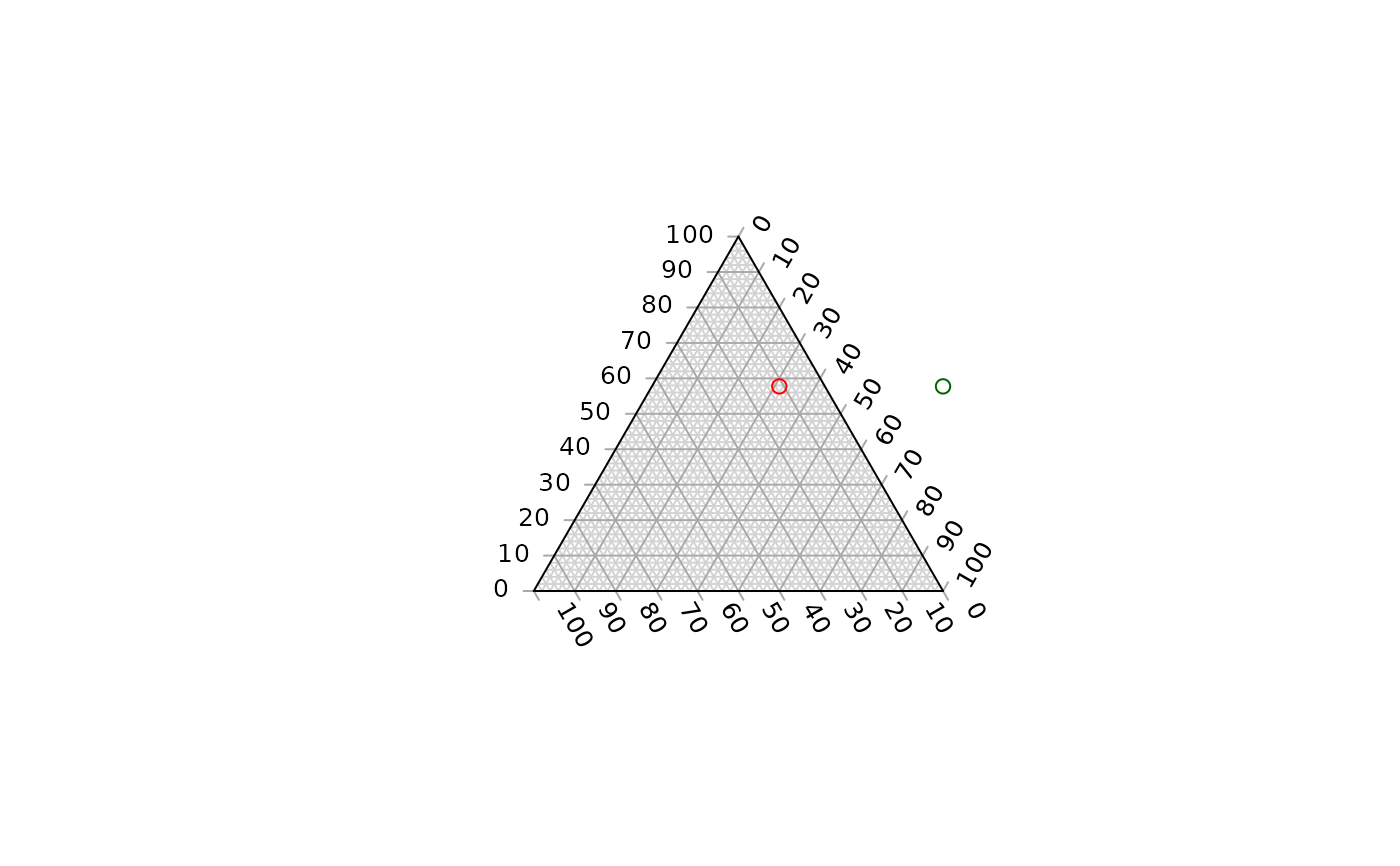Evaluate whether a given set of coordinates lie outwith the boundaries of a plotted ternary diagram.

OutsidePlot(x, y, tolerance = 0)

## Arguments

x, y

Vectors of x and y coordinates of points.

tolerance

Consider points this close to the edge of the plot to be inside. Set to negative values to count points that are just outside the plot as inside, and to positive values to count points that are just inside the margins as outside. Maximum positive value: 1/3.

## Value

OutsidePlot() returns a logical vector specifying whether each pair of x and y coordinates corresponds to a point outside the plotted ternary diagram.

Other plot limits: TernaryXRange()

## Examples


TernaryPlot()
points(0.5, 0.5, col = 'darkgreen')
OutsidePlot(0.5, 0.5)
#>  TRUE

points(0.1, 0.5, col = 'red')OutsidePlot(0.1, 0.5)
#>  FALSE

OutsidePlot(c(0.5, 0.1), 0.5)
#>   TRUE FALSE